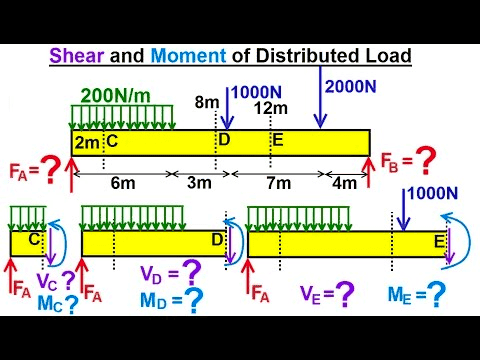# What is bending moment? Is it a moment couple? Why?

Leo Liu
In engineering statics I've learned the concept of bending moment, which in some way indicates an applied load that tends to bend the object, and it seems that it can move around freely in the reference frame. (I understand moment in engineering is not quite the same concept as torque in physics despite having the same unit.) For example, when finding the internal bending moment for a statically determined beam, we can often include directly the internal bending moment in the moment equation taken at the end of the beam. Is it really a free vector, which would thus suggest that it's a moment couple? Why is it so?

Last edited:

Homework Helper
Gold Member
… For example, when finding the internal bending moment for a statically determined beam, we can often include directly the internal bending moment in the moment equation taken at the end of the beam. Is it really a free vector, which would thus suggest that it's a moment couple? Why is it so?
Could you explain that portion a little better?
Perhaps an example?
Thank you.

Gold Member
I understand moment in engineering is not quite the same concept as torque in physics despite having the same unit.
According to Wikipedia:
https://en.wikipedia.org/wiki/Torque said:
In physics and mechanics, torque is the rotational equivalent of linear force. It is also referred to as the moment, moment of force, rotational force or turning effect, depending on the field of study.

Is it really a free vector, which would thus suggest that it's a moment couple? Why is it so?
In statics, the net force must be zero since nothing moves (no acceleration). Therefore, any force must be balanced by an equal and opposite net force in the same direction. If these forces are some distance apart, they will create a moment that is referred to as a couple:
https://en.wikipedia.org/wiki/Couple_(mechanics) said:
In mechanics, a couple is a system of forces with a resultant (a.k.a. net or sum) moment but no resultant force.
So yes, it is a free vector.

••Leo Liu and berkeman
Leo Liu
Could you explain that portion a little better?
Perhaps an example?
Thank you.In this example (random question found on Google), if we wanted to express the total moment of the leftmost beam about A (where F_A acts), the moment equation would be $$M=0=M_c-2V_C-400\cdot 1$$, even though M_C doesn't act directly at A.

Homework Helper
Gold Member
Where Fa acts, there is no internal moment, if the support is a pivot.
From that point, moving toward the middle point of the beam, the value of the internal moment or couple increases.

Mentor
It's not a couple. In the "Strength of Materials" approach to beam bending, we assume that all cross sections of the beam remain flat, and that the axial component of strain is proportional to the distance above or below the "neutral axis" within the beam. So the axial stress is zero at the neutral axis, and for downward bending, the axial strain and stress are in tension above the neutral axis and in compression below the neutral axis. The bending moment at a given cross section of the beam is equal to the integral over the cross section of the axial stress times the distance from the neutral axis. This can be replaced by a couple, but is certainly not fundamentally a couple.

•Leo Liu and berkeman
Leo Liu
It's not a couple. In the "Strength of Materials" approach to beam bending, we assume that all cross sections of the beam remain flat, and that the axial component of strain is proportional to the distance above or below the "neutral axis" within the beam. So the axial stress is zero at the neutral axis, and for downward bending, the axial strain and stress are in tension above the neutral axis and in compression below the neutral axis. The bending moment at a given cross section of the beam is equal to the integral over the cross section of the axial stress times the distance from the neutral axis. This can be replaced by a couple, but is certainly not fundamentally a couple.
Thanks for your insight! I have thought about this explanation, but I wasn't to sure about it because of the internal normal force. It makes sense to me now.

•berkeman
•